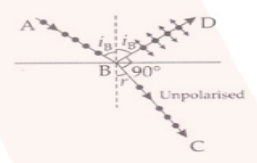# Can reflection result in plane-polarized light

Question:

Can reflection result in plane-polarized light if the light is incident on the interface from the side with higher refractive index?

Solution:When the angle of incidence is equal to the Brewster’s angle such that the transmitted light is polarized and reflected light is plane-polarized. Following is the equation:

$\operatorname{tani}_{B}=\mu_{2}^{1}=\frac{\mu_{2}}{\mu_{1}}$

Such that μ2 < μ1

When the light travels in a medium, the critical angle is given as

$\operatorname{sini}_{C}=\frac{\mu_{2}}{\mu_{1}}$

Where μ2 < μ1

So, it can be said that |tan iB| > |sin iC| when iB < iC.